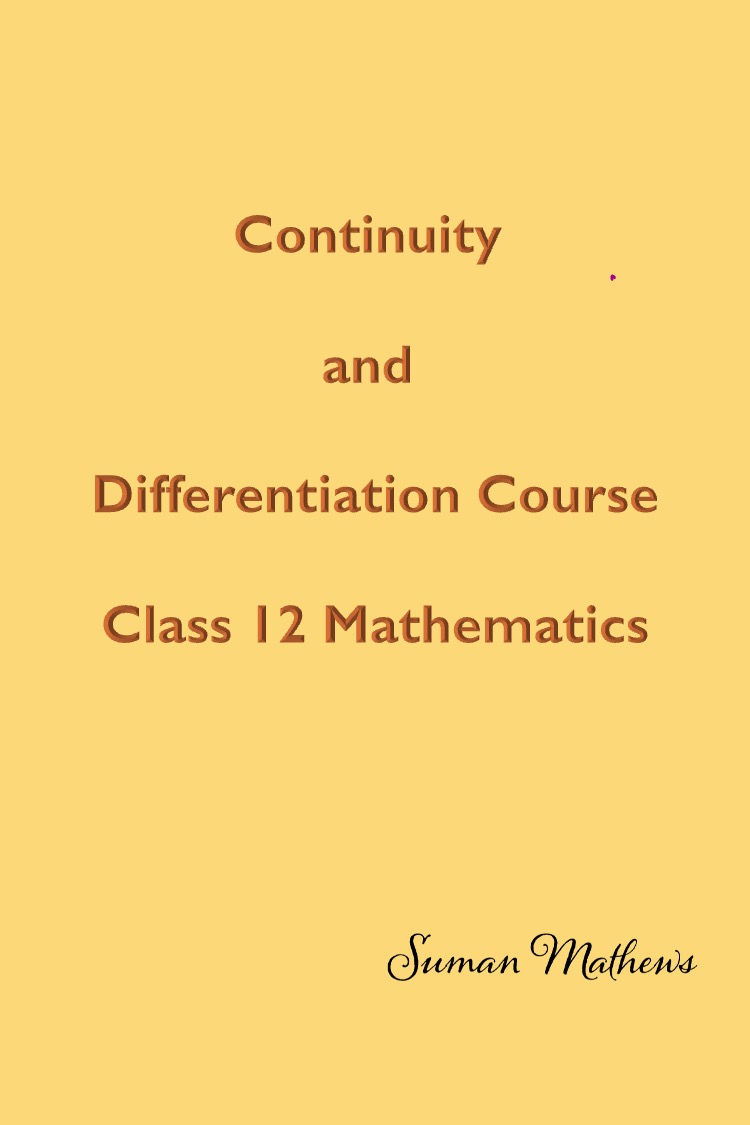top of page
Search

# Foundation Course in Continuity and Differentiability-Mathematics

Updated: Nov 10Here's embarking on a journey to the fascinating world of Differential Calculus. Let's start with a question: Imagine you are driving a car and you want to know how fast you're going at a given instant. One of my student's Sarah answered the question. ​ ​ She said that you need to know the rate at which you are travelling at a given instant. So, basically that is the essence of Differential Calculus. So let's dive into Derivatives- the fundamental tool for calculating the rate of change. ​ ​ Derivatives is used in real life scenarios such as tracking the growth of population, modeling the motion of objects and understanding the behaviour of functions. As you dive into this page, you'll find yourself immersed in a world of mathematical elegance. ​ ​ You'll solve problems that develop your analytical skills and appreciate the profound beauty of Differential Calculus. You'll develop the ability to solve particularly tricky questions that require a deeper understanding. Thus, the legacy of Differential Calculus continues inspiring you to embrace the power of mathematical thinking and explore the endless possibilities that lie ahead.

Has Differential Calculus baffled you? Hi and Welcome to Differentiation Part 2. I am Suman Mathews.

I have a double Master's in Mathematics and I've taught Maths for three decades at the high school and college level. Trust me. I know exactly what it takes to boost up your math scores. I have explained the basics of Differentiation in Part 1 of Differentiation.

If you like you can enrol for Part 1 of he course. it's free!

This course is a your first step to understanding Differential Calculus. This would greatly benefit students preparing for ISC and CBSE Class 12 Mathematics.

The course starts with

1. An introductory video which also includes all the formulas learnt in this session.

2. The concept of Continuity at a point and continuity in an interval.

3. You will learn how to calculate the left hand and right hand limits for continuity .

4. The concept of derivative at point using limits is illustrated.

5. You will learn how to calculate the derivative using definition.

6. You will then learn how to differentiate using the formula.

7. The course concludes with how to calculate the derivative of composite functions and using the Chain Rule.

You learn how to apply Chain Rule in a simple way. Moving on, you'll learn about how to incorporate differentiation of Inverse Trigonometric functions. The trick here, is to substitute and make the function simple.

A number of problems have been incorporated here and solved for your understanding. You'll learn what are Implicit Functions and how to differentiate them. The trick here is to just differentiate both sides of the equation.

Implicit Differentiation is explained quite in detail here. There is also an Assignment and a small test on multiple choice questions on what you've learnt so far. I hope this has served your purpose of learning Differentiation.

Get inspired and enrol for this course. Remember, a journey of a thousand miles begins with one step! Thank you!

You start with learning how to differentiate composite functions. This is an extremely important concept for you to master. Progressing through the course, you'll learn Implicit functions next.

So, what exactly is an Implicit function? How do you differentiate between an explicit and implicit function? Learn all this and how to differentiate Implicit Functions here.

Moving on, you learn about logarithmic differentiation.Given a function to the power of another function, a simple trick to follow will be to apply log to both sides. You then differentiate both sides, using the concept learnt in Implicit Differentiation.

You'll progress to learn how to differentiate Determinants. This can be done in two ways, either using row wise or column wise differentiation. Another extremely important concept you'll learn here is second order derivatives.

Learn what are second order derivatives and how to evaluate them. You'll also learn how to solve identities using second order derivatives. Formulas that you've learnt are also given to you as a special feature.

A good self learning course for you here. You can encourage your friends to join this course. Join my road map for differentiation. Enrol for this course